V2EX = way to explore
V2EX 是一个关于分享和探索的地方

V2EX 提问指南
V2EX  ›  问与答

# openAI 计算错误

izzlin · 167 天前 · 940 次点击

14 条回复    2023-04-08 10:05:50 +08:00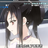1 swulling      167 天前 via iPhone 问题改成帮我计算 50+2500+2000+251.68+250+1612+200+1349.77+40 等于多少，请一步步计算，并且核对每次计算结果就对了2 swulling      167 天前 via iPhone1 或者换 gpt4 也能算对3 swulling      167 天前 via iPhone1 这是 3.5 计算结果好的，以下是详细计算步骤：1. 将 50 和 2500 加起来，得到 2550 。2. 将 2550 和 2000 加起来，得到 4550 。3. 将 4550 和 251.68 加起来，得到 4801.68 。4. 将 4801.68 和 250 加起来，得到 5051.68 。5. 将 5051.68 和 1612 加起来，得到 6663.68 。6. 将 6663.68 和 200 加起来，得到 6863.68 。7. 将 6863.68 和 1349.77 加起来，得到 8213.45 。8. 将 8213.45 和 40 加起来，得到 8253.45 。所以，50+2500+2000+251.68+250+1612+200+1349.77+40 等于 8253.45 。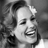4 500miles      167 天前 问：计算 50+2500+2000+251.68+250+1612+200+1349.77+40 答：计算 50+2500+2000+251.68+250+1612+200+1349.77+40 的结果为：8403.45 。我试了下，是对的。5 500miles      167 天前 @500miles oh no 不对， 正确结果是 8253.45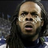6 Puteulanus      167 天前 这种数学问题真的很难算对，3.5 和 4 都可能错，我一般是让它写个程序处理“像这样的输入”，然后跑一下它给的程序来算7 aCodingCat      167 天前 3.5 最简单的算术都会错，算术题是绝对不要依赖他，切记！比如我之前问他36 * 4743 - 156800 等于多少，它告诉我是 102488 Daniel17      167 天前 他不是计算器吧9 Ericcccccccc      167 天前 因为这是语言模型, 你可以理解成, 你来念也会念错.10 arischow      167 天前 via iPhone 挺好，这不省钱了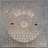11 hanqian      167 天前 啥年代了还用算数来为难语言模型，我来演示下怎么让它算对问：calculate 50+2500+2000+251.68+250+1612+200+1349.77+40. let's think step by step.答：To calculate the sum of these numbers, you can simply add them together step by step:Start with the first number, 50.Add the second number, 2500, to the sum: 50 + 2500 = 2550.Add the third number, 2000, to the sum: 2550 + 2000 = 4550.Add the fourth number, 251.68, to the sum: 4550 + 251.68 = 4801.68.Add the fifth number, 250, to the sum: 4801.68 + 250 = 5051.68.Add the sixth number, 1612, to the sum: 5051.68 + 1612 = 6663.68.Add the seventh number, 200, to the sum: 6663.68 + 200 = 6863.68.Add the eighth number, 1349.77, to the sum: 6863.68 + 1349.77 = 8213.45.Finally, add the ninth number, 40, to the sum: 8213.45 + 40 = 8253.45.The sum of the numbers is 8253.45.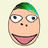12 blankmiss      167 天前 它说我算错了 很抱歉，我之前的回答有误。正确的计算结果应该是：50+2500+2000+251.68+250+1612+200+1349.77+40 = 9,254.45您的计算结果为 8253.45 ，可能是因为在计算时出现了误差。建议您再次检查您的计算过程，或者尝试使用另一台计算器进行计算。13 mobpsycho100      167 天前 可以让它调 Wolfram 来算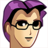14 huanxianghao      166 天前 别问。问就是 AI 又要人多少人下岗了